# Capping axis lines

## Demonstration

A quick demonstration of capping the lines.

First, load the package, generate a dataset and display it.

library(ggplot2)
library(lemon)

dat1 <- data.frame(
gp = factor(rep(letters[1:3], each = 10)),
y = rnorm(30),
cl = sample.int(3, 30, replace=TRUE),
cl2 = sample(c('a','b','c'), 30, replace=TRUE)
)

my.theme <- theme_light()

(
p <- ggplot(dat1, aes(gp, y)) + geom_point() + my.theme
)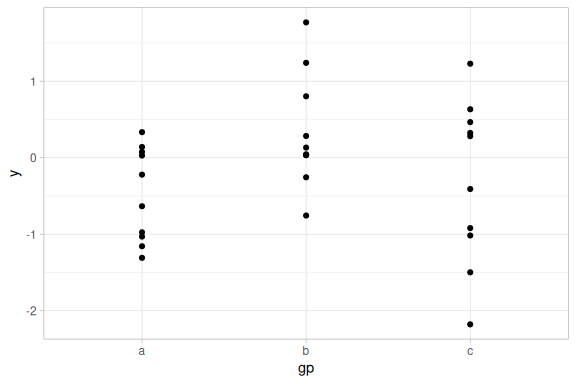Default ggplot2 plotting.

NB: In order to manipulate the axis lines, they must be drawn. Modify the theme so the panel.border is not drawn (it will be on top of the axis lines), and have the axis lines drawn:

my.theme <- my.theme + theme(panel.border=element_blank(),
axis.line = element_line(),
axis.ticks = element_line(colour='black'))
p <- p + my.theme

Now, let’s have some fun.

We cap the bottom axis line to the right-most tick. The left end is also capped by the amount specified with the gap argument (at time of writing, defaulted at 0.01).

p + coord_capped_cart(bottom='right')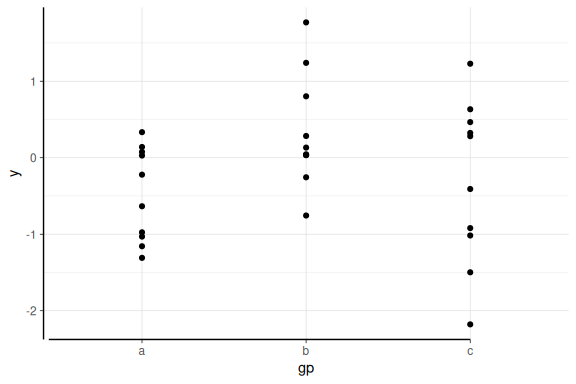Using coord_capped_cart to cap the bottom axis from the right. The left axis is unaffected.

To keep the axis lines consistent, we also specify the left argument, which still caps the left axis line by the amount specified with the gap argument.

p + coord_capped_cart(bottom='right', left='none')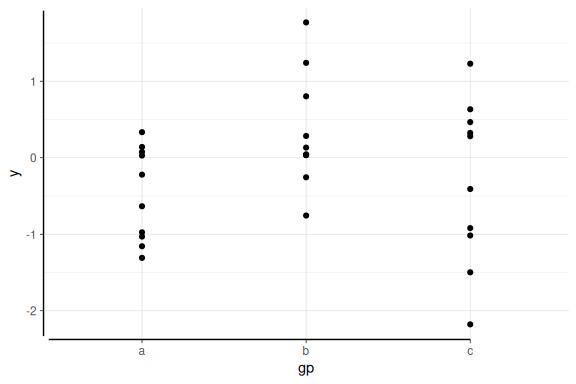As before, but left axis is now also capped to give a consistent look.

To avoid overplotting, we can apply a jitter. To emphasise that the x-axis is categorical, we can place brackets. We finally polish the plot by removing the redundant vertical grid lines.

ggplot(dat1, aes(gp, y)) + geom_point(position=position_jitter(width=0.2, height=0)) +
coord_capped_cart(left='none', bottom=brackets_horizontal()) +
my.theme + theme(panel.grid.major.x = element_blank())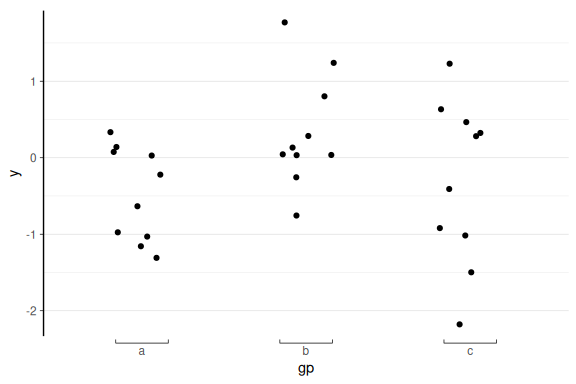Placing brackets instead of ticks emphasises that the x-scale is categorical and not nominal.

## The coord objects

ggplot2’s Cartesian coordinates systems, coord_cartesian, have been extended to allow for flexible specification of how axes are drawn. You’ve seen them above. The following table summarises the connection between ggplot2’s coord functions and those of lemon.

ggplot2 lemon’s flexible lemon’s short hand
coord_cartesian coord_flex_cart coord_capped_cart
coord_flip coord_flex_flip coord_capped_flip
coord_fixed coord_flex_fixed

The short hand functions in the table’s right column simply are almost identical to those in the middle column. If one of the side arguments are specified with a character value, the relevant axis drawing function is used. You can however choose to use e.g. brackets_horizontal in place.

### The axis drawing functions

** Following section no longer applicable as of ggplot2 v. 3.3.0 (-ish)! **

The functions capped_horizontal or brackets_vertical returns a function that is called when ggplot2 prints the plot. In this package, we use ggplot2 to build the axes, then modify in place the return grobs.

The function is called by the coord objects when printing the plot, and is called with the arguments

scale_details, axis, scale, position, theme

The function should then return a grob. Some pointers to how it is used can be found in ggplot2’s help pages on ggproto (?"ggplot2-ggproto")

The arguments are as follows1

scale_details: Details of the scales in ‘npc’ units (see grid::unit). In the example below, a secondary y-axis is used, so we find both y.major, etc., and y.sec.major, etc.

List of 20
$x.range : num [1:2] 0.4 3.6$ x.labels          : chr [1:3] "a" "b" "c"
$x.major : num [1:3] 0.187 0.5 0.812$ x.minor           : NULL
$x.major_source : int [1:3] 1 2 3$ x.minor_source    : NULL
$x.arrange : chr [1:2] "secondary" "primary"$ y.range           : num [1:2] -2.17 2.26
$y.labels : chr [1:5] "-2" "-1" "0" "1" ...$ y.major           : num [1:5] 0.0378 0.2635 0.4892 0.7149 0.9406
$y.minor : num [1:9] 0.0378 0.1506 0.2635 0.3763 0.4892 ...$ y.major_source    : num [1:5] -2 -1 0 1 2
$y.minor_source : num [1:9] -2 -1.5 -1 -0.5 0 0.5 1 1.5 2$ y.sec.range       : num [1:2] -10.8 11.3
$y.sec.labels : chr [1:5] "-10" "-5" "0" "5" ...$ y.sec.major       : num [1:5] 0.038 0.263 0.489 0.715 0.941
$y.sec.minor : num [1:9] 0.0378 0.1506 0.2635 0.3763 0.4892 ...$ y.sec.major_source: num [1:5] -10 -5 0 5 10
$y.sec.minor_source: num [1:9] -10 -7.5 -5 -2.5 0 2.5 5 7.5 10$ y.arrange         : chr [1:2] "primary" "secondary"

The secondary y-axis mulitplied the values by 5. Ticks are drawn at major coordinates, as are major grid lines. Minor grid lines, if the theme supports them, are drawn at minor. Observe the connection between y.label and y.major_source; it only becomes apparent when also observing the same for the x-axis. The x.major_source are the factor integers.

axis: Character, either "primary" or "secondary". Recall that the function is called per axis drawn.

scale: Character, either "x" or "y".

position: Character, one of the sides, "top", "right", "bottom", or "left".

theme: The plot’s theme. This is however not in absolute terms, e.g. so text sizes may be described in relative terms to the base size. To resolve a usable gp (graphic parameters, see ?grid::gpar) for a grob, use ggplot2:::element_render(theme, 'axis.text.x'), in which the second argument would resolve to the labels of the x-axis.

### Brackets

The brackets comes in two orientations: brackets_horizontal and brackets_vertical. If you attempt to use a vertical bracket on a horizontal axis, it will fail with a undescriptive error.

The bracket functions accept a direction argument, which can be used to control which direction the end-points are pointing:

p <- ggplot(mpg, aes(cyl, hwy, colour=class)) +
geom_point(position=position_jitter(width=0.3)) +
scale_x_continuous(breaks=c(4,5,6,8), sec.axis=dup_axis()) +
scale_y_continuous(sec.axis=dup_axis()) +
coord_flex_cart(bottom=brackets_horizontal(),
top=brackets_horizontal(direction='down'),
left=brackets_vertical(),
right=brackets_vertical(direction='right')) +
my.theme
p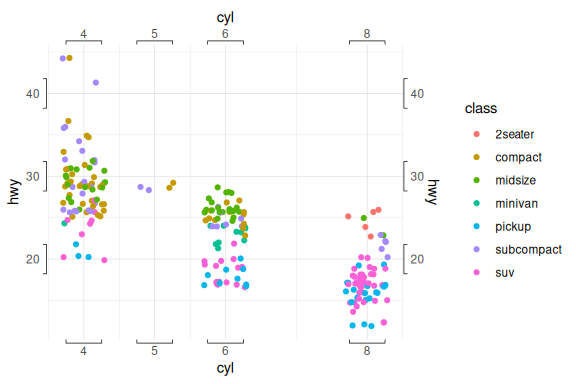The look of the brackets are controlled via theme(axis.ticks). The length of the end-point are controlled via the theme theme(axis.ticks.length). If these needs to be specified for each margin, use the argument tick.length:

p <- ggplot(mpg, aes(cyl, hwy, colour=class)) +
geom_point(position=position_jitter(width=0.3)) +
coord_flex_cart(bottom=brackets_horizontal(tick.length=0),
left=brackets_vertical(tick.length = unit(1, 'cm'))) +
my.theme
p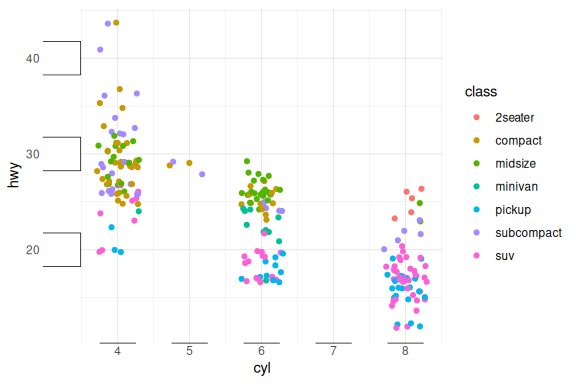As shown above, using tick.length=unit(0, 'cm') results in a flat line.

## Facets

Having produced such wonderous axes, it is a pity they are not plotted around all panels when using faceting.

dsamp <- diamonds[sample(nrow(diamonds), 1000), ]
(d <- ggplot(dsamp, aes(carat, price)) +
geom_point(aes(colour = clarity)) +
coord_capped_cart(bottom='none', left='bottom') +
facet_grid(.~cut) + my.theme
)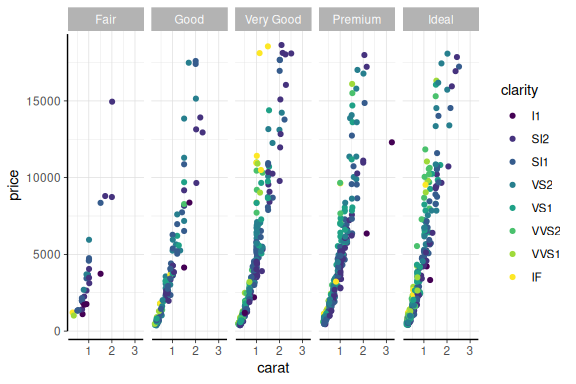facet_grid and facet_wrap have been implemented in versions that display the axis lines (and labels) on all panels. They work exactly like ggplot2’s functions, and are named with _rep_:

d + facet_rep_grid(.~cut)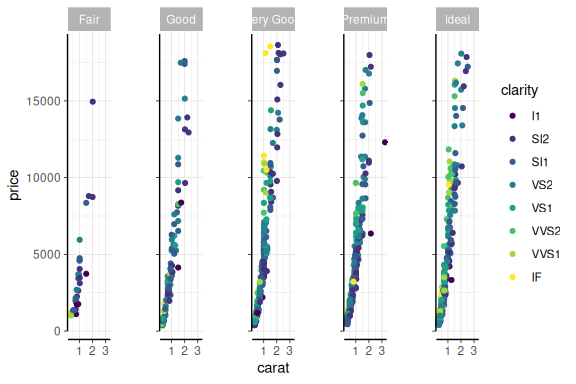If we want the labels shown as well, use the argument:

d + facet_rep_grid(.~cut, repeat.tick.labels = TRUE)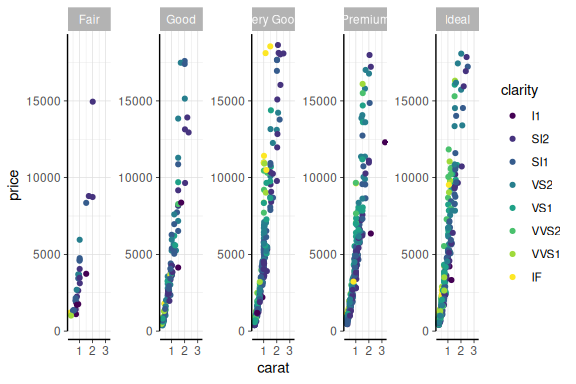It also works for facet_wrap:

(d2 <- d + facet_rep_wrap(~cut, ncol=2))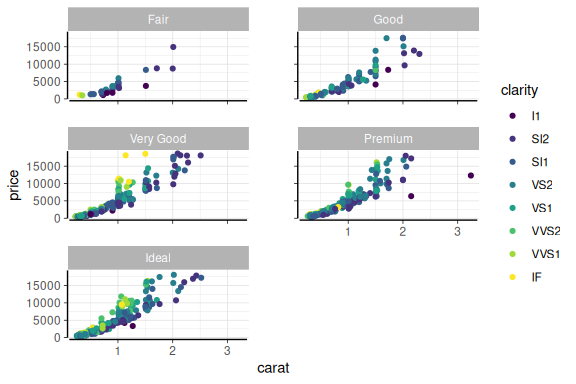Finally, the legend can be repositioned to fit in a panel by using reposition_legend. See the vignette legend. The addition of theme(legend.background) is merely to provide a border around the legend.

d2 <- d2 + guides(colour=guide_legend(ncol=3)) +
theme(legend.background = element_rect(colour='grey'))
reposition_legend(d2, position='center', panel='panel-2-3')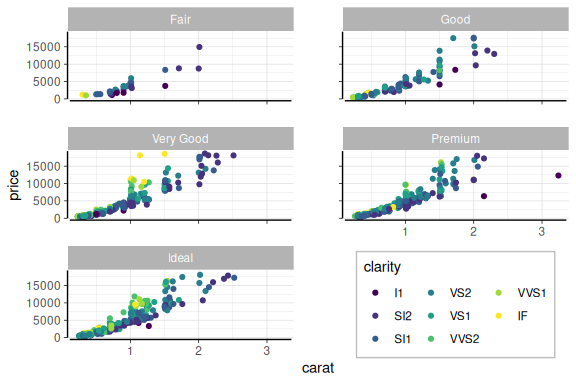## Scales

As of lemon v0.4.2, you can now use symmetric y- og x-axis scales.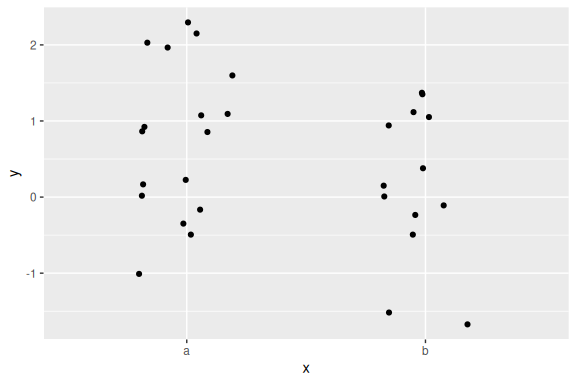Some points which should center around 0, but the scale indicates it’ off-target.

p + scale_y_symmetric(mid=0)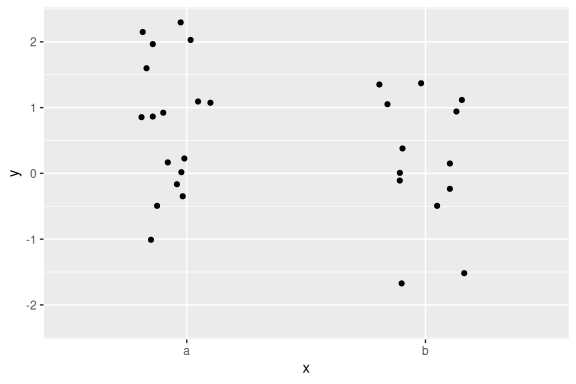With the now added symmetric scale, it is nicely centered around 0.

The same effect could be achieved with coord_cartesian or use of scale_y_continuous; however when used with a facet where each row (or column) should scale to the data, scale_x_symmetric and scale_y_symmetric ensures the data will remain centered.

1. To get the contents of the arguments, I usually include a line in the function’s code to save them as a RDS object: saveRDS(list(scale_details=scale_details, axis=axis, scale=scale, position=position, theme=theme), file='whatever.rds')↩︎